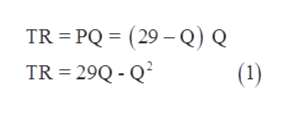# A monopolist’s cost function yields constant average and marginal costs, with AC = MC = 5. Thefirm faces a market demand curve given by P = 29 – Q.1. Calculate the marginal revenue curve, MR, for the monopoly.2. Solve for the monopolist’s profit maximizing output and price and calculate the Lerner’s index and the profit of the monopolist. Show the profit maximizing decision of the monopolist and the profits earned diagrammatically.3.  What would be the socially optimal output and price? (Hint: this would be the output and price if the industry were perfectly competitive. That is, the profit maximizing rule would be P =MC)4. Show diagrammatically the social cost of a monopoly. and Calculate the social cost of monopoly

Question
2 views
A monopolist’s cost function yields constant average and marginal costs, with AC = MC = 5. Thefirm faces a market demand curve given by P = 29 – Q.
1. Calculate the marginal revenue curve, MR, for the monopoly.
2. Solve for the monopolist’s profit maximizing output and price and calculate the Lerner’s index and the profit of the monopolist. Show the profit maximizing decision of the monopolist and the profits earned diagrammatically.
3.  What would be the socially optimal output and price? (Hint: this would be the output and price if the industry were perfectly competitive. That is, the profit maximizing rule would be P =MC)
4. Show diagrammatically the social cost of a monopoly. and Calculate the social cost of monopoly
check_circle

Step 1

Hi there! Thankyou for the question. Since we only answer up to 3 sub-parts, we will answer the first three. Please resubmit the question and specify the other sub-parts (up to 3) you would like to be answered.

Step 2

Given information,

Step 3

The total revenue can be calculated by multiplying price with quantity. Thus, multiply th...help_outlineImage TranscriptioncloseTR = PQ = (29 – Q) Q TR = 29Q - Q² (1) fullscreen

### Want to see the full answer?

See Solution

#### Want to see this answer and more?

Solutions are written by subject experts who are available 24/7. Questions are typically answered within 1 hour.*

See Solution
*Response times may vary by subject and question.
Tagged in